Nets
SA
Volume
Missing Dimension
Variety
100

My net is made up of six squares.

What is a cube?
100What is 150 cm2

100
Calculate the volume of the cube.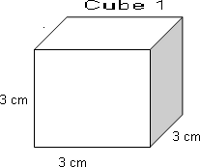What is 27 cm3?

100

The volume of a rectangular prism is 340 cm3. The length is 10 cm and the width is 2 cm. What is the height?

What is 17 cm?
100
Find the surface area of this net.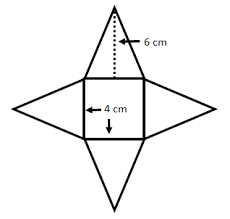What is 64 cm2?

200
My net is made up of one rectangle and four triangles.

What is a rectangular pyramid?

200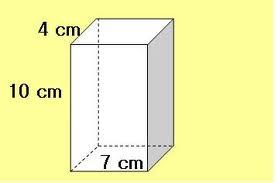What is 276 cm2

200
Calculate the volume of the rectangular prism.What is 120 cm3?

200

The volume of a rectangular prism is 819 cm3. The length is 13 cm and the width is 7 cm. What is the height?

What is 9 cm?
200
How many cubes are at the bottom of this rectangular prism?What is 15?
300My net is made up of two triangles and three rectangles.
What is a triangular prism?
300

Find the surface area of the following triangular pyramid. Each triangle is congruent.What is 84 ft2?

300
Calculate the volume of the cube.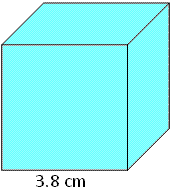What is 54.872 cm3?

300

The volume of a rectangular prism is  3135 cm3. The length is 15 cm and the height is 11 cm. What is the width?

What is 19 cm?
300
How many cubes does this rectangular prism hold? (Find the Volume)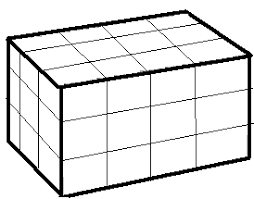What is 36 units3?

400
Name the netWhat is a triangular pyramid?
400

The world’s largest candy bar is a huge rectangular prism and weighs more than a ton! The bar is 10 feet long, 6 feet tall and 2 feet wide. What is the surface area of the candy bar?

What is 184 ft2?

400
Calculate the volume of the rectangular prism.What is 126 7/8 in3?

400

The volume of a rectangular prism is 5400 cm3. The length is 25 cm and the width is 18 cm. What is the height?

What is 12 cm?
400
Find surface area of the triangular prism.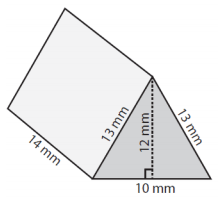What is 624 mm2?

500
My net is made up of two pentagons and five rectangles.
What is a pentagonal prism?
500What is 544 cm2?

500
A storage room measures 7 1/2 feet by 4 feet by

7 1/2 feet. What is the volume of the storage room?

What is 225 feet3 ?

500

The volume of a rectangular prism is 59,211 cm3. The length is 43 cm and the width is 27 cm. What is the height?

What is 51 cm?
500
What is the surface area of the triangular prism?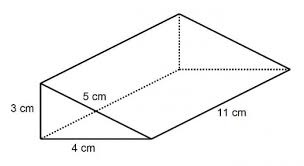What is 144 cm2?

Click to zoom